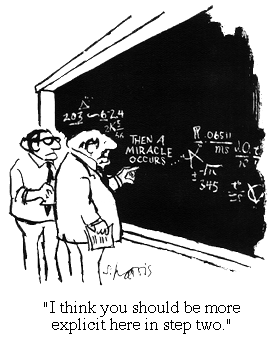## 2020\21 Ratings Calculations### So How Did I Get That Rating?

BAL ratings are calculated after each round.

•       Games won by forfeit are not counted for rating purposes.

•       For rating purposes, new, unrated players are assumed to have an initial rating of 1300 based on zero previous games.
The rating calculation formulas are modified versions of the old USCF ratings formulas.  There are two main calculation formulas: provisional, for players who have played fewer than 20 rated BAL games at the start of the rating cycle, and established, for those who have played 20 or more rated BAL games at the start of the rating cycle.

Within a rating cycle, performance ratings are first calculated for all new, unrated players.  These performance ratings are then used when calculating the ratings of the remaining players.

### Provisional Ratings Calculation

The provisional rating calculation formula is:

 (np × Rp) + ( ∑ Ro ) + (400 × ( ∑ W - ∑ L)) G  = np + nc -  Rp

where
G is the gain in rating (can be negative),
np is the number of games played prior to the ratings cycle,
Rp is the player's previous rating,
∑ Ris the sum of the ratings of the player's opponents,
∑ W is the sum of the player's wins (win = 1, draw = 0.5),
∑ L is the sum of the player's losses (loss = 1, draw = 0.5), and
nc is the number of games played in this ratings cycle

### Established Ratings Calculation

The established rating calculation is a bit more complex:

 G  = k × ( ∑W - ∑We )

where
G is the gain in rating (can be negative),
k is a constant based on the player's previous rating (16 if over 2399, 24 if  between 2100 and 2399, and 32 otherwise),
∑W is the sum of the player's game scores (win = 1, loss = 0, draw = 0.5), and
∑We is the sum of the player's "expected wins" against each opponent, computed for each opponent as:

 1 We  = 1 + 10 - (Δr ÷ 400)

where Δr is the difference between the player's and opponent's rating.

After the appropriate calculation is performed,  the resulting gain may be adjusted if the player's new rating crosses one of the "k" boundaries as follows ( RO = old rating, RN= new rating):

If (either RO or RN) > 2099
If ((both RO and RN) ≥ 2400) OR (2100 ≤ (both RO and RN) ≤ 2400)
RN is not changed
ElseIf (RO < 2100)
RN = 2100 + (RN - 2100) × 0.75
ElseIf RO < 2400
If RN < 2100
RN = 2100 + (RN - 2100) × 1.33
ElseIf RN > 2399
RN = 2400 + (RN - 2400) × 0.66
Else
RN is not changed
ElseIf RO ≥ 2400
If RN < 2400
RN = 2400 + (RN - 2400) × 1.50
Else
RN is not changed
Else
RN is not changed

### Rounding

Finally, if the change is less than 1, the new rating is rounded up (to the next highest integer) if G is positive, or rounded down (to the next lower integer) if G is negative.  This ensures that a positive result will mean the gain of at least one point, and a negative result will mean the loss of at least one point.